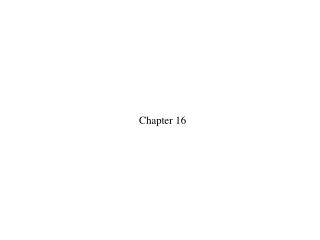DownloadDownload PresentationChapter 16

Chapter 16

Télécharger la présentationChapter 16

- - - - - - - - - - - - - - - - - - - - - - - - - - - E N D - - - - - - - - - - - - - - - - - - - - - - - - - - -
Presentation Transcript

1. Chapter 16

2. The pressure in a system is measured to be 60 kPa. At a later time the pressure is 40 kPa. The value of ∆p is 1. –40 kPa. 2. –20 kPa. 3. 0 kPa. 4. 20 kPa. 5. 40 kPa.

3. The pressure in a system is measured to be 60 kPa. At a later time the pressure is 40 kPa. The value of ∆p is 1. –40 kPa. 2. –20 kPa. 3. 0 kPa. 4. 20 kPa. 5. 40 kPa.

4. Which system contains more atoms: 5 mol of helium (A = 4) or 1 mol of neon (A = 20)? 1. Helium. 2. Neon. 3. They have the same number of atoms.

5. Which system contains more atoms: 5 mol of helium (A = 4) or 1 mol of neon (A = 20)? 1. Helium. 2. Neon. 3. They have the same number of atoms.

6. The temperature of a glass of water increases from 20°C to 30°C. What is ∆T? 1. 303 K 2. 293 K 3. 283 K 4. 10 K

7. The temperature of a glass of water increases from 20°C to 30°C. What is ∆T? 1. 303 K 2. 293 K 3. 283 K 4. 10 K

8. For which is there a sublimation temperature that is higher than a melting temperature? 1. Carbon dioxide 2. Water 3. Both 4. Neither

9. For which is there a sublimation temperature that is higher than a melting temperature? 1. Carbon dioxide 2. Water 3. Both 4. Neither

10. You have two containers of equal volume. One is full of helium gas. The other holds an equal mass of nitrogen gas. Both gases have the same pressure. How does the temperature of the helium compare to the temperature of the nitrogen? 1. Thelium < Tnitrogen 2. Thelium = Tnitrogen 3. Thelium > Tnitrogen

11. You have two containers of equal volume. One is full of helium gas. The other holds an equal mass of nitrogen gas. Both gases have the same pressure. How does the temperature of the helium compare to the temperature of the nitrogen? 1. Thelium < Tnitrogen 2. Thelium = Tnitrogen 3. Thelium > Tnitrogen

12. What is the ratio Tf /Ti for this process? 1. 4 2. 2 3. 1 (no change) 4. 5.

13. What is the ratio Tf /Ti for this process? 1. 4 2. 2 3. 1 (no change) 4. 5.